• 产品展示
•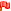磁翻板液位计磁浮球液位计浮球液位开关、控制器法兰式液位变送器智能电容式液位计投入式液位计、变送器雷达液位计玻璃管液位计锅炉双色水位计电磁流量计涡街流量计液体涡轮流量计超声波流量计孔板流量计金属管浮子流量计
• 全国销售热线：400-9280-163
电话：86 0517-86917118
传真：86 0517-86899586
销售经理：1560-1403-222 (丁经理)
139-1518-1149 (袁经理)
业务QQ：2942808253 / 762657048
网址：https://www.harzkj.com磁翻板液位计说明书选型液位变送器说明书选型浮球式液位计说明书选型投入式液位计说明书选型雷达液位计说明书选型射频导纳液位计说明书选型重锤式料位计说明书选型电容式液位计说明书选型浮标液位计说明书选型浮球液位开关说明书选型电磁流量计说明书选型超声波流量计说明书选型涡轮流量计说明书选型涡街流量计说明书选型金属管流量计说明书选型V锥流量计说明书选型孔板流量计说明书选型楔形流量计说明书选型

# 安装位置的不同会对双法兰差压式液位计参数设置造成什么影响

发表时间：2020-04-02   点击次数：  技术支持：15601403222

双法兰液位变送器，又被叫做双法兰差压液位计，实际上也是液位计的一种。它是由测量膜片与两侧绝缘片上的电极所组成的一个电容器，可用于测量液体、气体，或者蒸汽的压力、流量、甚至密度；是一种安装于容器或者管道上的远传装置，也是一种可以防止管道中的介质直接进入变送器的压力传感器组件；它与变送器之间靠注满流体的毛细管而连接，当检测到压力货流量时，会将其转变成4～20mA DC的信号输出。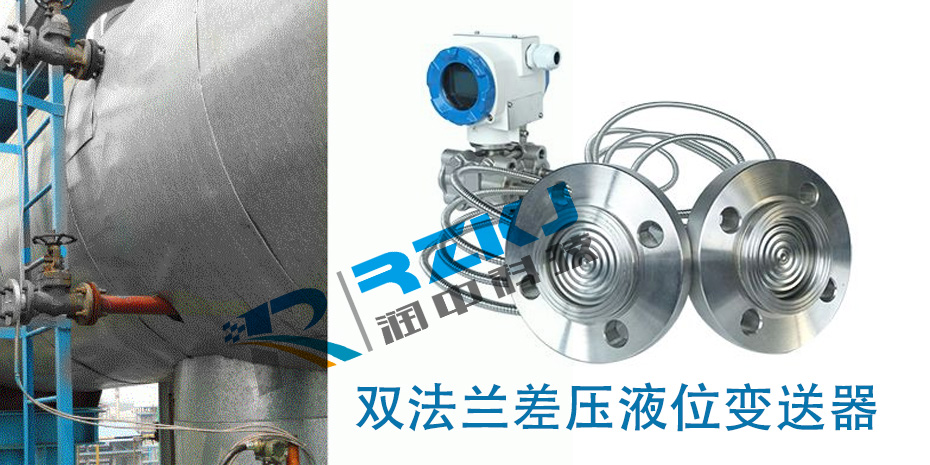1变送器低于上下法兰口

双法兰差压式液位计安装在密闭容器低端法兰水平线以下,变送器安装在密闭容器下法兰水平线以下, 如下图所示。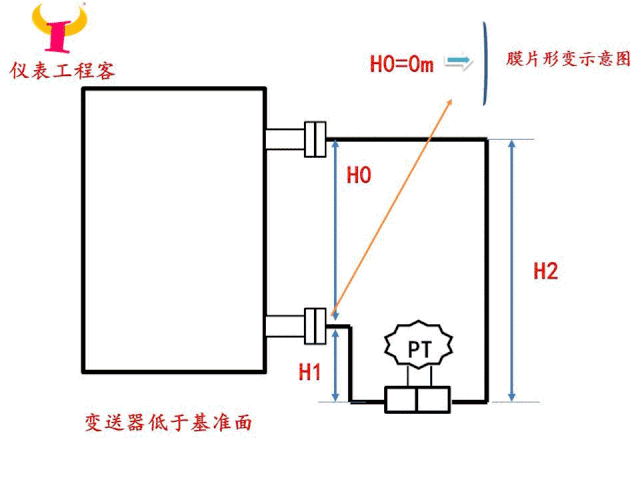假设ρ介为介质密度=1.5，ρ0为硅油密度=0.93，H0为介质量程0-5m,H1=1m，H2=6m，求变送器的量程。

解：

量程：ΔP=ρ介×g×H0=1.5×9.8×5=73.5KPa

高压侧空液位压力：P（+）=ρ0×g×H1=0.93×9.8×1=9.114KPa

低压侧空液位压力：P（-）=ρ0×g×H2=0.93×9.8×6=54.684KPa

空液位时压力差：ΔP= P（+）- P（-）=9.114-54.684=-45.57KPa

满液位时压力差：ΔP=空液位压力差ΔP+量程值ΔP=-45.57+73.5=27.93KPa

变送器的量程为：-45.57至27.93KPa

2变送器位于上下法兰之间

双法兰差压式液位计安装在密闭容器法兰水平线中间,变送器安装在密闭容器高低侧法兰水平线中间,如下图所示。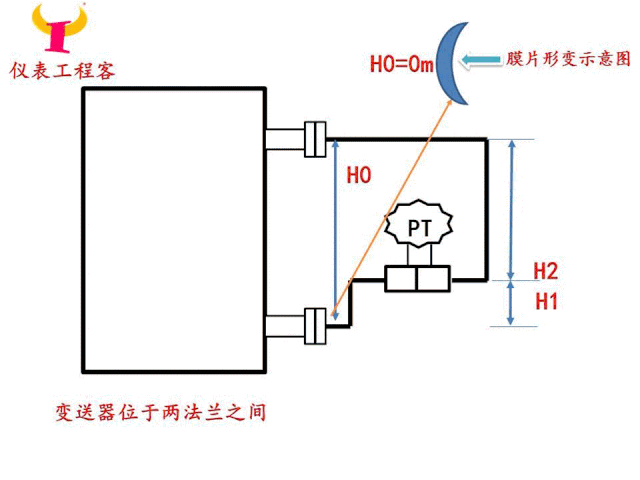假设ρ介为介质密度=1.5，ρ0为硅油密度=0.93，H0为介质量程0-5m,H1=2m，H2=3m，求变送器的量程。

解：

量程：ΔP=ρ介×g×H0=1.5×9.8×5=73.5KPa

高压侧空液位压力：P（+）= ρ0×g×-H1=0.93×9.8×-2=-18.228KPa

低压侧空液位压力：P（-）=ρ0×g×H2=0.93×9.8×3=27.342KPa

空液位时压力差：ΔP= P（+）- P（-）=-18.228-27.342=-45.57KPa

满液位时压力差：ΔP=空液位压力差ΔP+量程值ΔP=-45.57+73.5=27.93KPa

变送器的量程为：-45.57至27.93KPa

3变送器高于上下法兰

双法兰差压变送器安装在密闭容器高侧法兰水平线以上,如下图所示。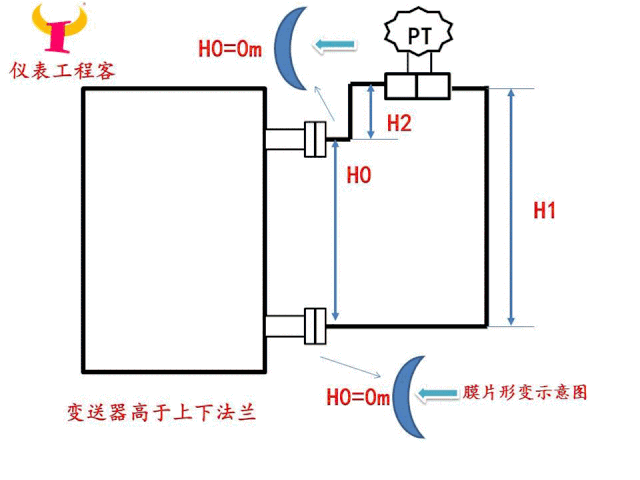假设ρ介为介质密度=1.5，ρ0为硅油密度=0.93，H0为介质量程0-5m,H1=6m，H2=1m，求变送器的量程。

解：

量程：ΔP=ρ介×g×H0=1.5×9.8×5=73.5KPa

高压侧空液位压力：P（+）= ρ0×g×（-H1）=0.93×9.8×-6=-54.684KPa

低压侧空液位压力：P（-）=ρ0×g×（-H2）=0.93×9.8×-1=-9.114KPa

空液位时压力差：ΔP= P（+）- P（-）=-54.684-（-9.114）=-45.57KPa

满液位时压力差：ΔP=空液位压力差ΔP+量程值ΔP=-45.57+73.5=27.93KPa

变送器的量程为：-45.57至27.93KPa

结论

在仪表施工与调试当中，经常有同仁讲以上三种安装方式零点迁移量是不一样的。从上面的计算公式中可以得出，双法兰差压式液位计变送器不管安装在什么位置，其量程和零点迁移量不会随变送器安装位置改变而改变。实际安装中为了变送器能使用更长久，推荐***种安装方法。

相关文章

分享到：